# eryar

posts - 409, comments - 576, trackbacks - 0, articles - 0

# OpenCASCADE解非线性方程组

eryar@163.com

Abstract. 在科学技术领域里常常提出求解非线性方程组的问题，例如，用非线性函数拟合实验数据问题、非线性网络问题、几何上的曲线曲面求交问题等。OpenCASCADE中有关于非线性方程组定义的类及其求解类，本文主要介绍如何在OpenCASCADE中定义非线性方程组，及对其进行求解。

Key Words. Function Set, Function Set Root, Newton Raphson Algorithm

### 1.Introduction

OpenCASCADE提供了非线性方程组的类math_FunctionSet，可以先从类图上来看看有哪些算法使用了这个类：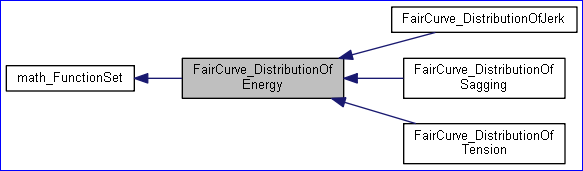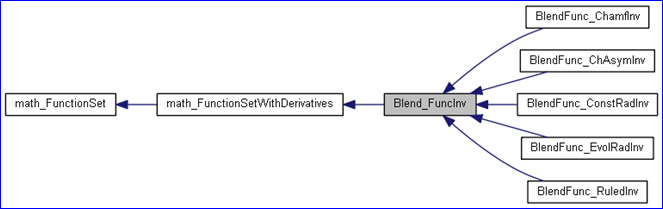### 2.Function Set Definition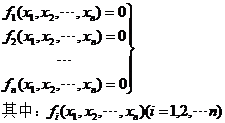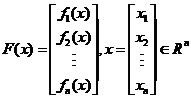OpenCASCADE中使用类math_FunctionSet来表示方程组，这是个抽象类，定义了如下纯虚函数：

l NbVariables()：变量的个数，即末知量的个数；

l NbEquations()：方程的个数，即方程组中有几个方程；

l Value(const math_Vector&X, math_Vector& F)：方程组的值，即代入变量每个方程的值；

### 3.Function Set Root Algorithm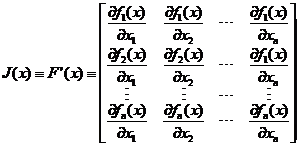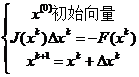OpenCASCADE中也提供了非线性方程组的求解类，如：math_FunctionSetRootmath_NewtonFunctionSetRoot。而使用这些类的输入都是要求具有一阶偏导数的线性方程组的定义math_FunctionSetWithDerivaties。这个类定义了具有一阶偏导数的非线性方程组，其纯虚函数除了前面说明的几个以外，还增加了如下两个：

l Derivatives(const math_Vector& X, math_Matrix& D)：一阶偏导数值，即计算Jacobi矩阵；

l Values(const math_Vector& X, math_Vector& F, math_Matrix& D)：计算方程的值及一阶偏导数矩阵Jacobi矩阵。

### 4.Code Example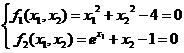#include <math_FunctionSet.hxx>
#include
<math_FunctionSetWithDerivatives.hxx>
#include
<math_FunctionSetRoot.hxx>
#pragma comment(lib,
"TKernel.lib")
#pragma comment(lib,
"TKMath.lib")
class MyFunctionSet : public math_FunctionSetWithDerivatives
{
public:

virtual Standard_Integer NbVariables() const
{

return 2;
}

virtual Standard_Integer NbEquations() const
{

return 2;
}

virtual Standard_Boolean Value(const math_Vector& X, math_Vector& F)
{
F(
1= X(1* X(1+ X(2* X(2- 4.0;
F(
2= Pow(M_E, X(1)) + X(2- 1.0;

return Standard_True;
}

virtual Standard_Boolean Derivatives(const math_Vector& X, math_Matrix& D)
{

// matrix D is Jacobi matrix.
D(11= 2.0 * X(1);
D(
12= 2.0 * X(2);
D(
21= Pow(M_E, X(1));
D(
22= 1.0;

return Standard_True;
}

virtual Standard_Boolean Values(const math_Vector& X, math_Vector& F, math_Matrix& D)
{
Value(X, F);
Derivatives(X, D);

return Standard_True;
}
private:
};
void test()
{
MyFunctionSet aFunctionSet;
math_FunctionSetRoot aSolver(aFunctionSet);
math_Vector aStartingPoint(
12);

// 1. (1.0, 1.0)
aStartingPoint(1= 1.0;
aStartingPoint(
2= 1.0;
aSolver.Perform(aFunctionSet, aStartingPoint);

if (aSolver.IsDone())
{
aSolver.Dump(std::cout);
}

// 2. (1.0, -1.0)
aStartingPoint(1= 1.0;
aStartingPoint(
2= -1.0;
aSolver.Perform(aFunctionSet, aStartingPoint);

if (aSolver.IsDone())
{
aSolver.Dump(std::cout);
}
}
int main(int argc, char* argv[])
{
test();

return 0;
}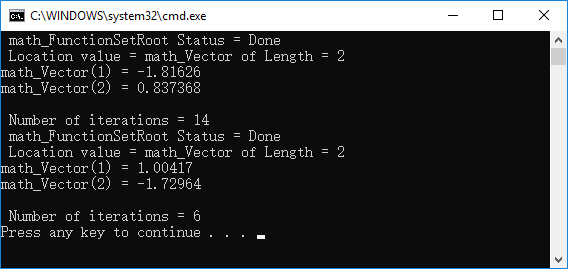### 5.Conclusion

OpenCASCADE类图中可以看出，方程组定义类用在了很多地方，所以理解上述对方程组的定义及解的用法，对其他使用这个派生类的地方更容易其源码。

### 6.References

1. 同济大学数学教研室高等数学 第四版高等教育出版社. 2004
2. 易大义沈云宝李有法计算方法浙江大学出版社. 2002

##### 为了方便大家在移动端也能看到我的博文和讨论交流，现已注册微信公众号，欢迎大家扫描下方二维码关注。只有注册用户登录后才能发表评论。 【推荐】超50万行VC++源码: 大型组态工控、电力仿真CAD与GIS源码库 相关文章: